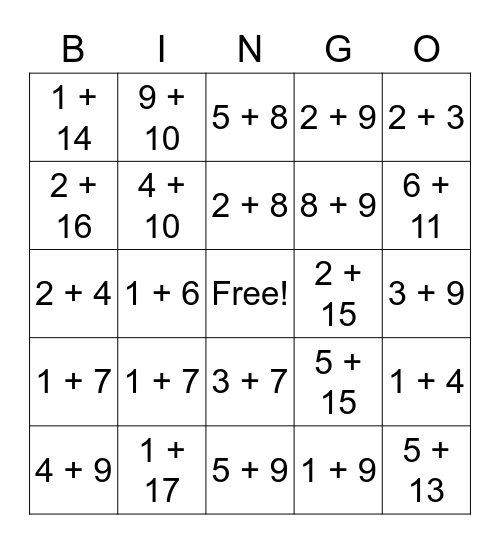# Addition to 20 Bingo!This bingo card has a free space and 124 words: 1 + 1, 1 + 2, 1 + 3, 1 + 4, 1 + 5, 1 + 6, 1 + 7, 1 + 8, 1 + 9, 1 + 10, 1 + 11, 1 + 12, 1 + 13, 1 + 14, 1 + 15, 1 + 16, 1 + 17, 1 + 18, 1 + 19, 2 + 2, 2 + 3, 2 + 4, 2 + 5, 2 + 6, 2 + 7, 2 + 8, 2 + 9, 1 + 1, 1 + 2, 1 + 3, 1 + 4, 1 + 5, 1 + 6, 1 + 7, 1 + 8, 1 + 9, 1 + 10, 1 + 11, 1 + 12, 1 + 13, 1 + 14, 1 + 15, 1 + 16, 1 + 17, 1 + 18, 1 + 19, 2 + 2, 2 + 3, 2 + 4, 2 + 5, 2 + 6, 2 + 7, 2 + 8, 2 + 9, 2 + 10, 2 + 11, 2 + 12, 2 + 13, 2 + 14, 2 + 15, 2 + 16, 2 + 17, 2 + 18, 3 + 3, 3 + 4, 3 + 5, 3 + 6, 3 + 7, 3 + 8, 3 + 9, 3 + 10, 3 + 11, 3 + 12, 3 + 13, 3 + 14, 3 + 15, 3 + 16, 3 + 17, 4 + 4, 4 + 5, 4 + 6, 4 + 7, 4 + 8, 4 + 9, 4 + 10, 4 + 11, 4 + 12, 4 + 13, 4 + 14, 4 + 15, 4 + 16, 5 + 5, 5 + 6, 5 + 7, 5 + 8, 5 + 9, 5 + 10, 5 + 11, 5 + 12, 5 + 13, 5 + 14, 5 + 15, 6 + 6, 6 + 7, 6 + 8, 6 + 9, 6 + 10, 6 + 11, 6 + 12, 6 + 13, 6 + 14, 7 + 7, 7 + 8, 7 + 9, 7 + 10, 7 + 11, 7 + 12, 8 + 8, 8 + 9, 8 + 10, 8 + 11, 9 + 9, 9 + 10 and 10 + 10.

⚠ This card has duplicate items: 1 + 1 (2), 1 + 2 (2), 1 + 3 (2), 1 + 4 (2), 1 + 5 (2), 1 + 6 (2), 1 + 7 (2), 1 + 8 (2), 1 + 9 (2), 1 + 10 (2), 1 + 11 (2), 1 + 12 (2), 1 + 13 (2), 1 + 14 (2), 1 + 15 (2), 1 + 16 (2), 1 + 17 (2), 1 + 18 (2), 1 + 19 (2), 2 + 2 (2), 2 + 3 (2), 2 + 4 (2), 2 + 5 (2), 2 + 6 (2), 2 + 7 (2), 2 + 8 (2), 2 + 9 (2)

## Play Online